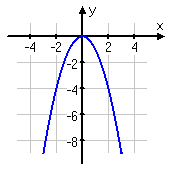Effects of "a"
Reflecting Over the x-axis

Another effect of "a" is to reflect the graph across the x-axis. When the parent function f(x) = x2 has an a-value that is less than 0, the graph reflects across the x-axis before it is transformed. The graph below represents the function f(x) = -x2.In function notation, this reflection is represented by a negative outside the function: -f(x). If the negative is inside the function notation, there is a reflection across the y -axis. Since a parabola is already symmetric about the y -axis, this transformation does not actually change the look of the parabola.

Listen